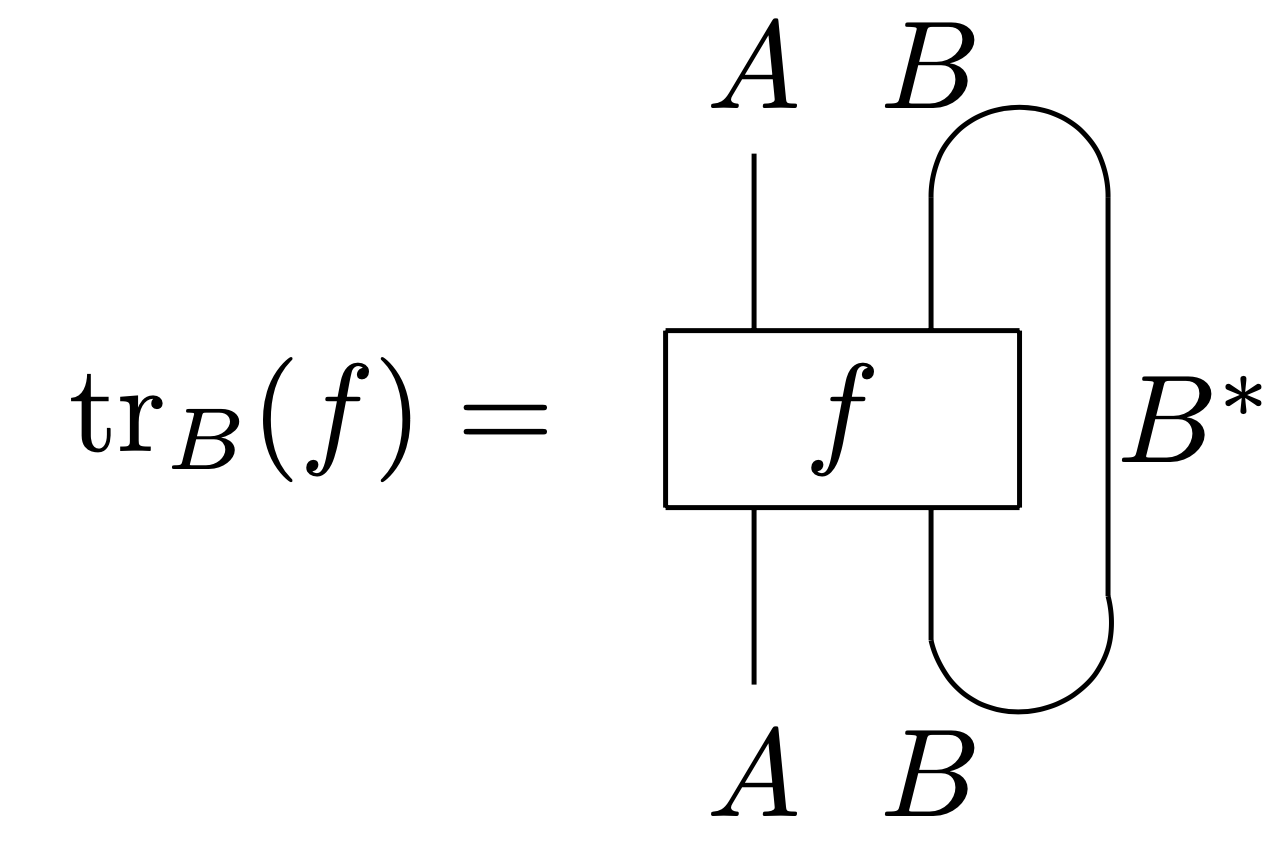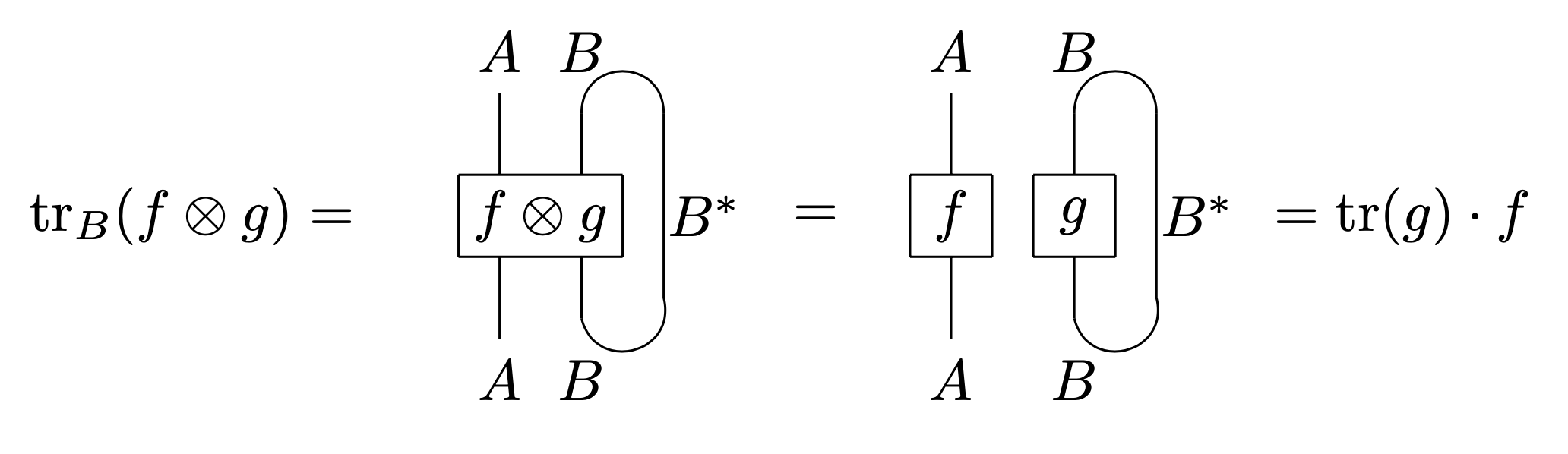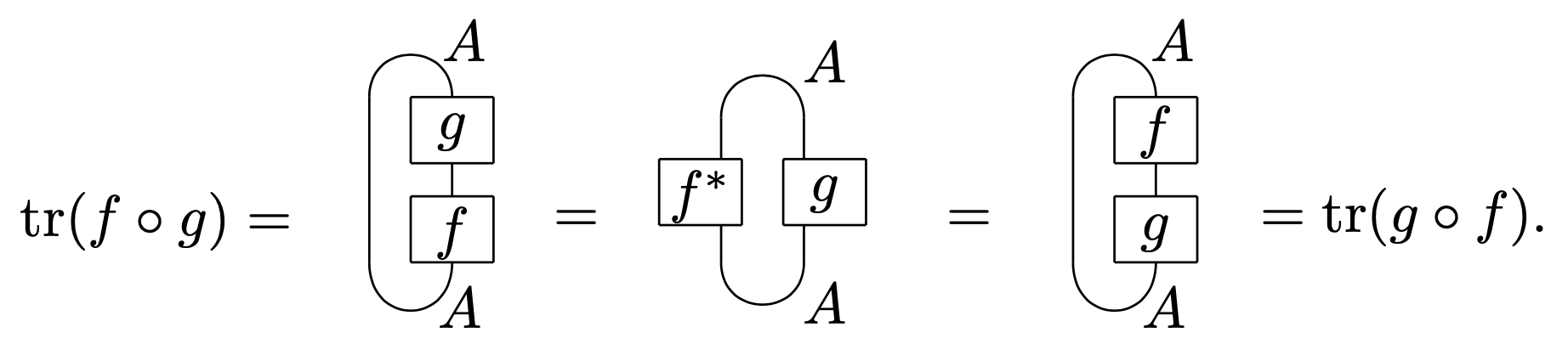Contents

(…)

# Contents

## Idea

A partial trace is a trace applied to (only) one factor in a tensor product.

The partial trace is generalized by traced monoidal categories. That is, a traced monoidal category is a monoidal category paired with an operation which behaves like the partial trace.

## Definition

Let $\text{Vec}_k$ denote the category of finite-dimensional vector spaces over a field $k$. The partial trace gives a compatible collection of linear maps

$\tr_W \colon \text{End}(V\otimes W)\to \text{End}(V)$

given by

$\tr_W(f\otimes g) \,=\, \tr(g)\cdot f \,,$

where “$tr$” on the right denotes the usual trace.

Explicitly, the partial trace can also be defined as follows:

Let $f \colon V\otimes W\to V\otimes W$ be an endomorphism. Let $v_{1}, \ldots, v_{m}$ and $w_{1}, \ldots, w_{n}$ be linear bases for $V$ and $W$ respectively. Then $f$ has a matrix representation $\{a_{kl,ij}\}$ where $1 \le k,i \le m$ and $1 \le l,j \le n$ relative to the basis of the space $V \otimes W$ given by $e_{k} \otimes f_{l}$. Consider the sum

$b_{k,i} \,=\, \sum_{j=1}^{n}a_{k j, i j}$

for $k,i$ over $1, \ldots, m$. This gives the matrix $b_{k,i}$. The associated linear operator on $V$ is independent of the choice of bases and corresponds to the partial trace.

## String diagram representations

Given vector spaces $A,B$, or more generally, given objects $A,B$ in a traced monoidal category, we will often write the partial trace using string diagrams as follows:Considering $\text{Vec}_k$ as a pivotal category, this diagram is completely rigorous. To make sure this notation is consistent, we verifyAdditionally the closed loop can only be trace. This is because the axioms of a pivotal category we can move around the loop, soIt is a standard fact from linear algebra that the trace is uniquely characterized by the fact that it is linear and acts the same on $f\circ g$ and $g\circ f$ for all $f,g:A\to A$.

Last revised on September 24, 2023 at 09:56:40. See the history of this page for a list of all contributions to it.